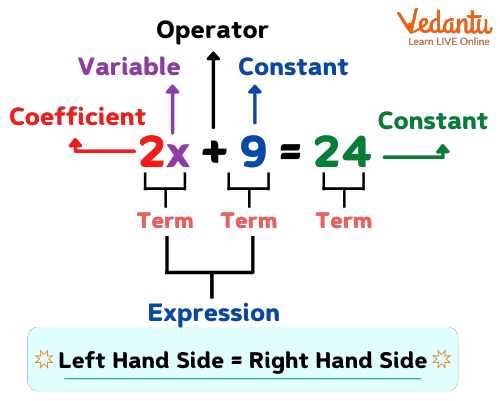Courses
Courses for Kids
Free study material
Free LIVE classes
More

# Equations ExamplesLIVE
Join Vedantu’s FREE Mastercalss

## What are Equations in Math?

Equations are something that we can understand as the combination of numbers, operators on the left hand side and the result on the right hand side separated by an equal to ‘=’ sign’. The operators that we study in math are +, -, , andFor example, my mom brought two chocolates of Dairy milk and three 5-star chocolates for my younger sister, so how many chocolates does my sister have? Well, here, we will be using the addition (+) operator because the total is being asked here.

2 + 3 = 5

So, the numbers on adding give 5 as the result on the right-hand side. This is how we understand equations in math. Here, on this page, we will go through certain more equation examples.

## Certain Examples for Equations in Math

Example 1: Yesterday, Shrishti purchased apparel worth Rs. 350. Today, she decided to buy another dress but before that she has to count how much she is left with. Like earlier, she had Rs. 1000 in her pocket, so which approach she follows to determine the amount she has at present?

Answer: Well, it is so easy to determine the remaining amount so that she could decide either to buy a new dress or not.

So, original amount she had = Rs. 1000

Amount she spent yesterday = Rs. 350

Now, the amount she has left with is ‘?’

So, 350 + ? = 1000

Here, when we take 350 on the right-hand side, the sign before it changes from ‘+’ to ‘-’, so we have:

? = 1000 - 350

Please note that  the process of moving a term from one side of the equation to the other side and changing the sign before the term is transposition.

And, now using the concept of borrowing (subtraction regrouping), we have:

Here, 5 is greater than 0, so we borrow ‘1’ from the next place value, which is again ‘0’ and then again we go the other digit at the next place value, we find ‘1’ there. Now, when we take ‘1’ from here, the number in the minuend becomes 10 which is greater than ‘5’ in the subtrahend, so we get 10 - 5 = 5.

Also, earlier in one’s place, we had 0 - 0, which was clearly ‘0’.

So, last two digits becomes ‘50’, now moving to the next digit, which after giving ‘1’ to the minuend corresponding to ‘5’ in the subtrahend becomes ‘9’ in place of ‘10’, so now we have a new minuend corresponding to ‘3’ as 9 and subtracting 9 from 3, i.e., 9 - 3 = 6.

Now, our answer becomes 650. This means that Shrishti is left with Rs. 650 after purchasing apparel of Rs. 350 yesterday.

Example 2: Suppose your brother’s height is three times your height and this is just an assumption, means that you are not aware of your height. Now, if you subtract ‘5’ from your brother’s height and suppose that 13 is the result, how do you interpret this information in an equation form?

Answer: Well, it is pretty simple to understand and follow the underlines steps:

Step: Assume that your height is ‘b’ cm and then your brother’s height becomes three times, i.e., ‘3b’ cm. And here you have assumed that the result is around 13 cm after subtracting ‘5’ from your brother’s height, so let us form an equation now:

3b - 5 = 13

3b = 13 + 5 (‘-’ sign on the LHS becomes ‘+’ when migrating to the right-hand side)

3b = 18

b = 18

So, b =  6 cm

Hence, your interpretation for the height turns out to be a single digit number, i.e., your height is 6 cm.

From the above text, we understand that equations are statements of equality between two expressions which are composed of numbers, variables, and operators. Going through these examples will help you understand what equations are and how we solve them.

Last updated date: 28th Sep 2023
Total views: 141k
Views today: 1.41k

## FAQs on Equations Examples

1. What are the 4 types of equations?

The four types of equations are as follows:

• Linear Equation